# Chapter 1 Network Theorems - Notes, Network Theory, Electrical Engineering Electrical Engineering (EE) Notes | EduRev

## Electrical Engineering (EE) : Chapter 1 Network Theorems - Notes, Network Theory, Electrical Engineering Electrical Engineering (EE) Notes | EduRev

The document Chapter 1 Network Theorems - Notes, Network Theory, Electrical Engineering Electrical Engineering (EE) Notes | EduRev is a part of the Electrical Engineering (EE) Course Electrical Engineering SSC JE (Technical).
All you need of Electrical Engineering (EE) at this link: Electrical Engineering (EE)

NETWORK THEOREMS

1. Thevenin's theorem
2. Norton's theorem
3. Superposition theorem
4. Reciprocity theorem
5. Max power transfer theorem
6. Compensation theorem
7. Tellegan's theorem
8. Milliman's theorem
9. Substitution theorem

1. Thevenin's Theorem: Any two terminal linear bilateral network can be replaced by a voltage source in series with an impedance. The voltage source is the voltage across the open circuited terminals and series impedance is the equivalent impedance seen across the terminals by replacing all the sources in network with their internal impedances.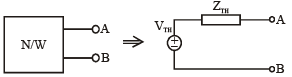VTH → Thevenin's voltage = VOC across AB.
ZTH → Thevenin's equivalent impedance

2. Norton's theorem: Any two terminal linear bilateral network containing active and passive elements can be replaced by an equivalent current source in parallel to an equivalent impedance current source is equal to short circuited current through the given terminals and equivalent impedance is the impedance seen across the terminals by replacing all the sources with their internal impedance.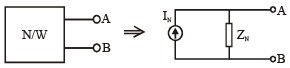IN → Norton's equivalent current
ZN → Norton's equivalent impedance.
ZN= ZTH
Here also, there can be three cases as in Thevenin's theorem. And, the equivalent ZTH calculation procedure remains same.

3. Superposition theorem: This theorem states that if a no. of voltage sources or current sources are acting together in an bilateral network then resultant current voltage in any branch is algebraic sum of current / voltage due to individual sources by replacing them with their internal impedance.

Note:
(a) The superposition theorem is applicable to linear time varying and LTI network.
(b) It is also applicable for circuit having initial condition.
(c) It is applicable for linear network.

Example: For the given circuit, the current I is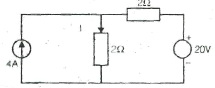(a) 2 A
(b) 5A
(c) 7 A
(d) 9A
Solution:
(c) Use superposition theorem.
Due to current source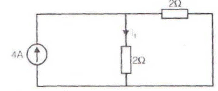I1 = (2/2+2).4 = 2A

Due to voltage source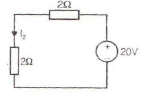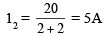Current I = I1 + I2
⇒ I = 2 + 5
⇒ I = 7A

4. Reciprocity Theorem: This theorem states that in a linear bilateral reciprocal network if I is the current in branch X due to a voltage source V in branch Y then same current will flow in branch Y when the same voltage source is connected in branch X.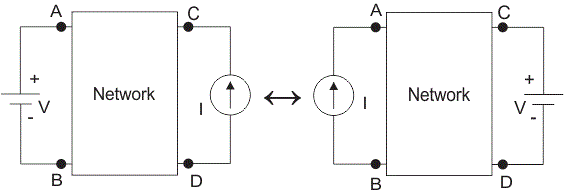Note:
(a) The initial conditions are assumed to be zero in reciprocity theorem.
(b) There should not be any extra dependent or independent sources in network.

5. Maximum Power Transfer Theorem: The following maximum power transfer theorems determine the values of the load impedance which results in maximum power transfer across the terminals of an active network. We consider a series combination of source and fixed complex impedance delivering power to a load consisting of a variable resistance or a variable  complex impedance.
Case 1: Load is variable resistance RL: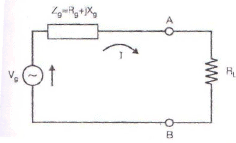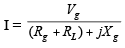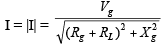Power delivered to RL is: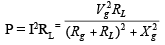To determine the value of R for maximum power transferred to the load,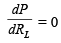We arrive at the condition: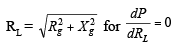Hence, with a variable pure resistance load the maximum power is delivered across the terminals of the active network if the load resistance is made equal to the absolute value of the active network impedance.
If the reactive component of the impedance in series with source is zero i.e., Xg = 0, then the maximum power is transferred to the load when the load and source resistances are equal i.e., RL = Rg.
Case 2: Load Impedance Zwith variable resistance and variable reactance: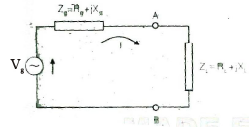For maximum power transfer: RL= Rg, XL = –Xg i.e. ZL = Zg

With the load impedance consisting of variable resistance and variable reactance, maximum power transfer across the terminals of the active network occurs when the load impedance Z1 is equal to the complex conjugate of the network impedance Zg.
Case 3: Load impedance, Zwith variable resistance and fixed reactance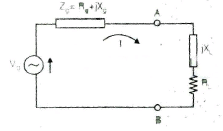For maximum power transfer.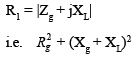6. Compensation Theorem: The compensation theorem is useful in determining the current and voltage changes in a circuit element when the value of its impedance is changed.
This application occurs in bridge and potentiometer circuits where a slight change in one impedance results in shift from the null condition.
Statement: In a linear time invariant bilateral network if the resistance R of a branch is changed by  ΔR then the currents in all branch of the network will change and this change in current can be found by connecting a voltage source VC such that VC = i ΔR  in series with resistance (R +ΔR ).
Here the current i represent current through R before change and all sources are replaced by their internal impedance to find out the change in current.

7. Tellegen's theorem: This theorem states that the algebraic sum of power consumed and delivered by each branch of a network is always zero.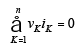n= no. of branches of network , v=voltage across Kth branch.

8. Millman's theorem: The Millman’s Theorem states that – when a number of voltage sources (V1, V2, V3……… Vn) are in parallel having internal resistance (R1, R2, R3………….Rn) respectively, the arrangement can replace by a single equivalent voltage source V in series with an equivalent series resistance R.  In other words; it determines the voltage across the parallel branches of the circuit, which have more than one voltage sources, i.e.,  reduces the complexity of the electrical circuit.

This Theorem is given by Jacob Millman. The utility of Millman’s Theorem is that the number of parallel voltage sources can be reduced to one equivalent source. It is applicable only to solve the parallel branch with one resistance connected to one voltage source or current source. It is also used in solving network having an unbalanced bridge circuit.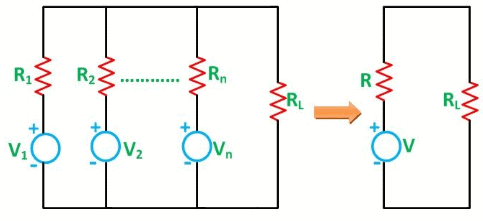As per Millman’s Theorem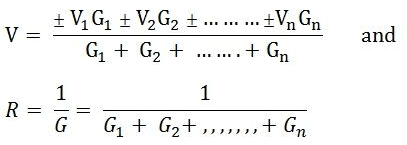9. Substitution theorem:

Statement: A known voltage in a branch can be replaced by an ideal voltage source.

• This theorem applies to any network, linear or non linear, time varying or time invariant.
• It is applicable both in the time domain and in the 's' domain.

Explanation of Substitution Theorem
Let us take a circuit as shown in fig – a,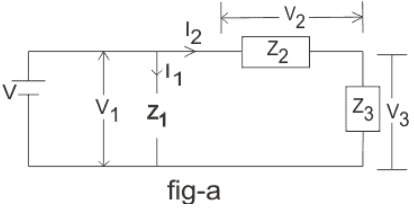Let, V is supplied voltage and Z1, Z2 and Zis different circuit impedances. V1, Vand V3 are the voltages across Z1, Z2 and Z3 impedance respectively and I is the supplied current whose I1 part is flowing through the Z1 impedance whereas I2 part is flowing through the Zand Z3 impedance.

Now if we replace Z3 impedance with V3 voltage source as shown in fig-b or with Icurrent source as shown in fig-c then according to Substitution Theorem all initial condition through other impedances and source will remain unchanged.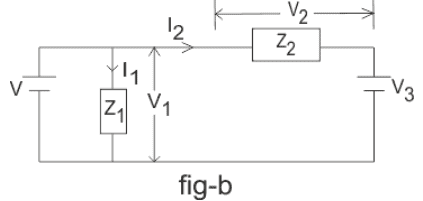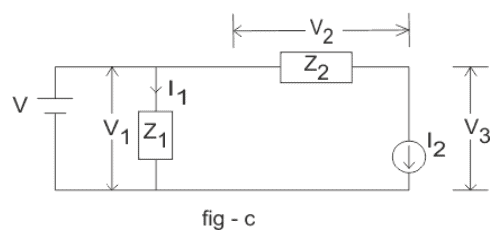i.e. – current through source will be I, voltage across Z1 impedance will be V1, current through Z2 will be I2 etc

Offer running on EduRev: Apply code STAYHOME200 to get INR 200 off on our premium plan EduRev Infinity!

78 docs|37 tests

,

,

,

,

,

,

,

,

,

,

,

,

,

,

,

,

,

,

,

,

,

,

,

,

,

,

,

;